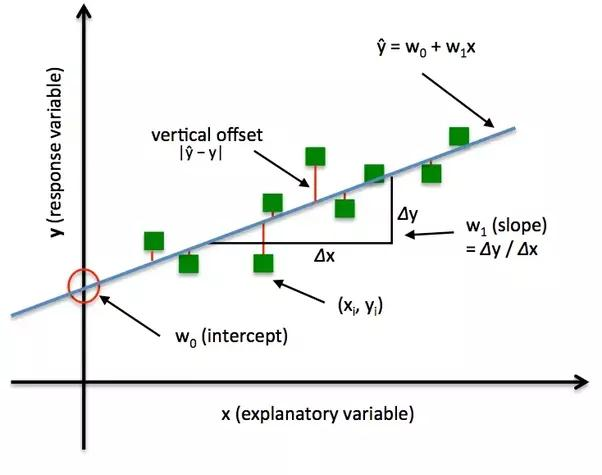/  Machine learning   /  Loss Functions in Machine Learning## Loss Functions in Machine Learning

In this article we will learn the Loss Functions, types and its applications.

Loss function is very simple, which is used to evaluate how well our algorithm works.if our predictions are totally deviate too much from the actual values the loss function would large number and vice versa. By using an optimization function the loss function learns to reduce the error in our prediction values.

Generally In machine learning models, we are going to predict a value given a set of inputs. The model has a set of weights and biases that you can tune based on a set of input data. The training data has several pairs of predicted and actual values.For this we use a loss function to determine how far the predicted values are  deviate from the actual values in the training data. We can update or change the model weights to make the loss minimum. In mathmatical notation the loss function will be

(Y_predicted – Y_actual)

Example:

If the predictions are too high or to low from the actual values it does not matter. The matter is how the predictions are incorrect.We are having different types of loss functions.

=>> Regression Losses.

=>> Classification losses

### 1. In Regression Losses:

1.Mean squared error.

2.Mean absolute error

3.Mean bias error.

### 2. In Classification Losses:

1.Hinge or svm  loss

2.Cross Entropy loss.

### 1. Mean squared error:

Mathematical formulation:As the name suggests, Mean square error is used to measure the average of squared difference between predictions and actual observations. It’s only consider the average magnitude of error irrespective of their direction.

```def MSE(y_predicted, y):

squared_error = (y_predicted - y) ** 2

sum_squared_error = np.sum(squared_error)

mse = sum_squared_error / y.size

return(mse)```### 2. Mean absolute error:

Mathematical formulation :-

a. Actual Costs – Let us assume the actual cost of houses are

2 bedroom — \$300K

3 bedroom — \$500K

4 bedroom — \$700K

b. Predicted Costs – Now, assume predicted cost of houses

2 bedroom — \$330K

3 bedroom — \$590K

4 bedroom — \$740K

Here the prediction error is

Prediction error = Actual – Predicted

For 2 bed room house

Error= actual – predicted.

Absolute Error 1 = |Error| (positive value of our error)

For 3 bed room house

Error= actual – predicted.

Absolute Error 2 = |Error| (positive value of our error)

For 4 bed room house

Error= actual – predicted.

Absolute Error 3 = |Error| (positive value of our error)

And n is the total number of training set.

n == 3

Mean Absolute Error = (Absolute Error 1 + Absolute Error 2 + Absolute Error 3) / nMean Absolute Error = (\$30K + \$90K + \$40K)/3

MAE = \$53.K

Mean absolute error, is measured as the average of sum of absolute differences between predictions and actual observations. It is very similar to MSE, measures the magnitude of error without considering their direction. The MAE is more robust to outliers so it does not make use of square.

### 3. Mean bias error:

Mathematical formulation :-The Mean bias error is very much less common in machine learning applications.This is also same as MSE but the only difference is  that we don’t take absolute values.so here to take a caution because the positive and and negative errors are cancel each other.this will effects the accuracy.

### 1. Hinge loss or Svm loss:

Mathematical formulation :-Hinge loss is used for the high maximum-margin classification which is nothing but the support vector machines.simply we can say that the score of correct category should be greater than sum of scores of all wrong categories by some safety margin.

### 2. Cross-Entropy loss:

Mathematical formulation :-This is the most widely used in classification problems.it increases the predicted probability. When the actual class is 0 the first half of function will disappears and the actual class is 1 the second half of function will disappears. The graph below is for true label is 1.